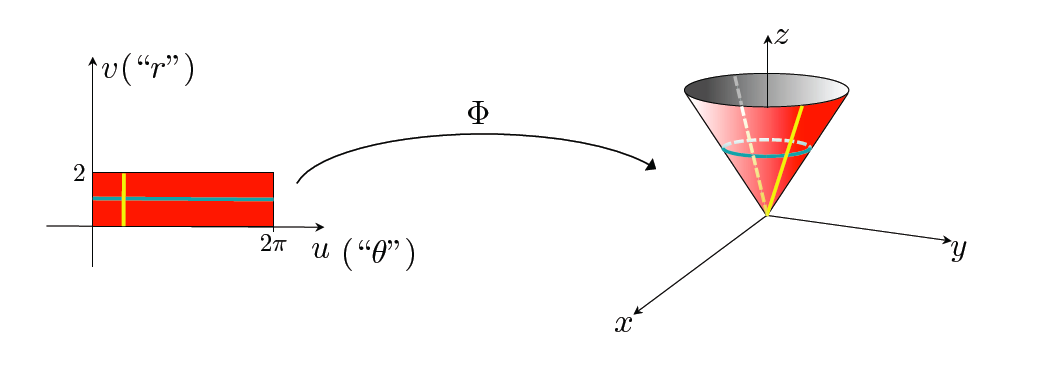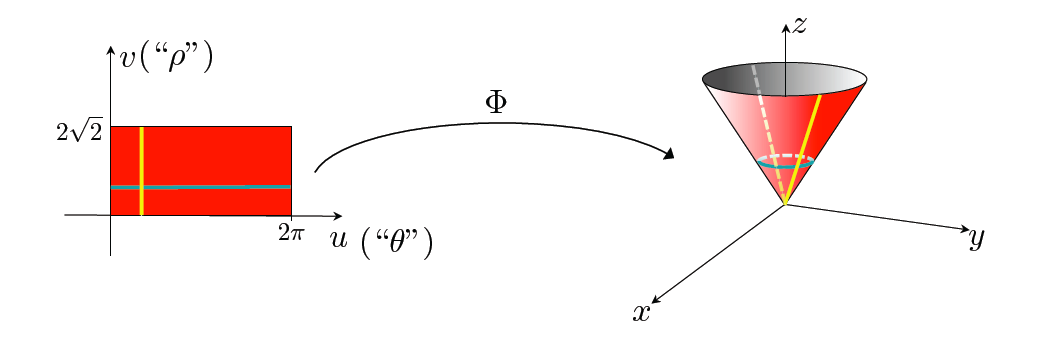## Parametrizing Surfaces

In analogy to parametrizing curves, where a curve is the image of a map of a straight interval in $\R^{1}$, a parametrized surface is the image of a map of a flat domain in $\R^{2}$.

Mathematically, a parametrized surface is the image of the map $\Phi : \mathcal{D} \subset \R^{2} \rightarrow \R^{3}$. The collection of points as $(u,v)$ varies in the domain $\mathcal{D}$ makes up the surface $\mathcal{S}$. The picture above happens to shows a rectangular domain, but this definition allows for any flat domain.

Expanded out in coordinates, we can write

$\mathcal{S}: \Phi(u,v) = (x(u,v), y(u,v), z(u,v)), (u,v) \in \mathcal{D}$

### Parametrizing using Other Coordinate Geometries

Before giving a number of examples of parametrized surfaces, we take a detour here to give an example of how a surface can be described using different coordinate geometries and how this affects the domain of the parametrization. In addition to showing that parametric descriptions of surfaces are not unique, this also highlights some of the advantages of using certain coordinate geometries, which can have computational advantages such as simplifying the domain of parametrization to a rectangular domain.

For this example, we will be taking the cone defined by the equation $z = \sqrt{x^2 + y^2}, z \leq 2.$Using each of the three coordinate geometries of cartesian, cylindrical, and spherical, we can write down three different parametric descriptions of this cone. We can further illustrate the difference between these descriptions by looking at the images of the constant lines $u = const, v = const$, which are called the isocurves of each parametrization.

Cartesian coordinates : Since this surface is a graph (which can be written as $z = f(x,y)$ and satisfies the "vertical line" test) we can assign $u = x , v = y$ so the parametrization is given by $\Phi(u,v) = (u, v, \sqrt{u^2 + v^2})$ and $\mathcal{D}$ is the disc of radius 2.Cylindrical coordinates : In cylindrical coordinates, this surface can be described by $z = r, 0 \leq r \leq 2, 0 \leq \theta \leq 2\pi$. The two variables that vary make up the domain, so we can set $u = \theta, v = r$. Expressing $x, y, z$ in terms of cylindrical coordinates, the parametrization is given by $\Phi(u,v) = (v \cos{u}, v \sin{u}, v)$, and $\mathcal{D}$ is the region where $0 \leq u \leq 2\pi$ and $0 \leq v \leq 2$ (which in shorthand can be written as $\mathcal{D} = [0, 2\pi]\times [0,2]$).Spherical coordinates : The description of this cone using spherical coordinates is given by $\phi = \pi/4, 0 \leq \rho \leq 2\sqrt{2}, 0 \leq \theta \leq 2\pi$. Again, the two variables that vary make up the domain, so we can assign $u = \theta, v = \rho$. The parametrization is then give by $\Phi(u,v) = (v \sin {\pi/4} \cos{u}, v\sin {\pi/4} \sin{u}, v\cos{\pi/4}) = (\frac{v}{\sqrt{2}} \cos{u}, \frac{v}{\sqrt{2}}\sin{u}, \frac{v}{\sqrt{2}})$ and $\mathcal{D} = [0, 2\pi] \times [0, 2\sqrt{2}]$.### Examples

You can play with any combination of functions $x(u,v), y(u,v), z(u,v)$ together with a fixed domain to create surfaces, but it is useful to have a working base of parametrizations of familiar surfaces that can be used as building blocks for your own creations.

Any graph given by $z = f(x,y)$ in a domain $(x,y) \in \mathcal{D}$ can be parametrized by setting $u = x, v = y$ which gives the parametrization

$\Phi(u,v) = (u, v, f(u, v)), (u,v) \in \mathcal{D}$

Graphs covers many surfaces, but is quite restrictive, even for the case of planes (since vertical planes are not graphs). We have already given a description of planes in terms of its normal vector, but we can also give a parametric description in terms of $\vec r_0$, which has its head on the plane and two vectors $\vec v_1, \vec v_2$ which lies in the plane. Given these quantities, we can write in vector notation

$\Phi(u,v) = \vec r_0 + u \vec v_1 + v \vec v_2$

Beyond graphs and planes, there are many useful non-graph surfaces that may result from folding, bending or twisting. One of the greatest benefits in parametrizing using other coordinate geometries is that we can parametrize many of these non-graph surfaces quite simply.

A helicoid can be parametrized as $\Phi(u,v) = (v \cos{u}, v\sin{u}, u)$ where $\mathcal{D} = [0, 2\pi] \times [r_1, r_2]$ and the internal and external radii satisfy $0 \leq r_1 < r_2$.

Any surface of revolution resulting from rotating a curve $r = f(z), z \in [a,b]$ about the z-axis can be conveniently described using cylindrical coordinates.Such a surface can be described by $r =f(z), 0 \leq \theta \leq 2\pi, a \leq z \leq b$. Setting $u = \theta, v = z$, the parametrization for this surface is $\Phi(u,v) = (f(v) \cos{u}, f(v) \sin{u}, v)$ and $\mathcal{D}$ is the rectangular domain $[0, 2\pi] \times [a,b]$. The function that determines a catenoid is $f(z) = c \cosh{\frac{z}{c}}$, and that for a hyperboloid is $f(z) = a \sqrt{1 + z^2}$.

In addition to surface variations that result from transforming these surfaces by a rigid motion, there are surfaces such as an elliptic hyperboloid for which the parametric description can be deduced readily as $\Phi(u,v) = (k_1\sqrt{1 + v^2} \cos{u}, k_2\sqrt{1 + v^2} \sin{u}, k_3 v)$ and $\mathcal{D} = [0, 2\pi] \times [a,b]$.